# Calculating a Project’s End Date in Microsoft Excel 2010

In this article, we will learn how to calculate a project’s end date in Microsoft Excel 2010.

Consider you are handling 10 projects at a time. While preparing reports, you are required to track the end date of the project. To calculate the end date, we will use Workday function.

Workday: Returns the serial number of the date before or after a specified number of workdays.Working days exclude weekends and any holidays dates. WORKDAY function can be used for calculating due dates, expected delivery times or the number of days of work performed. It calculates from start date to end date.

Syntax: =WORKDAY(start_date,days,holidays)

start_date: It is the date that represents the start date.

days: Days here refer the number of weekdays counted to get the end date. A positive value in days arguments will return a future date while a negative value will return a past date.

holidays: This is an optional argument. This will include the predefined holiday dates to be excluded from the working calendar.

Let us take an example:

• In column A, we have Start Date, and column B contains Days.
• In column C, we want to find out the end date of the project.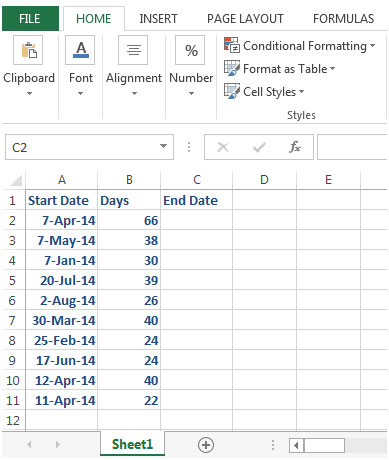• To calculate the work days, we will enter the formula in cell C2=WORKDAY(A2,B2)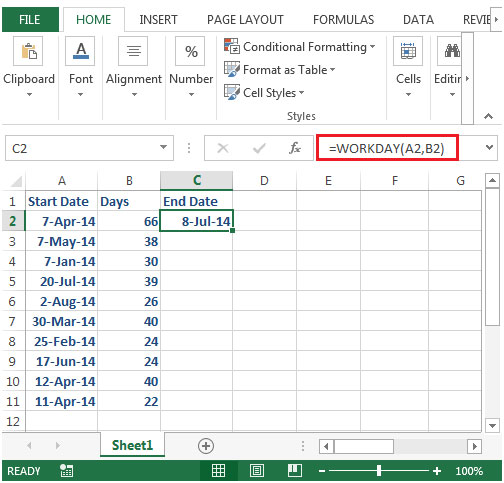• Copy the formula from cell C2 to range C3:C11, and you will the desired output.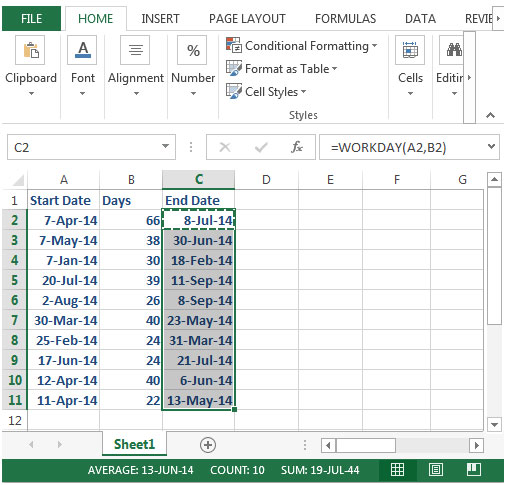• In this example, we have not used the third optional argument i.e. holidays
• Let us see the results after using holidays argument. We have a list in column F & G.
• Enter the workday formula in cell D2=WORKDAY(A2,B2,\$F\$3:\$F\$10)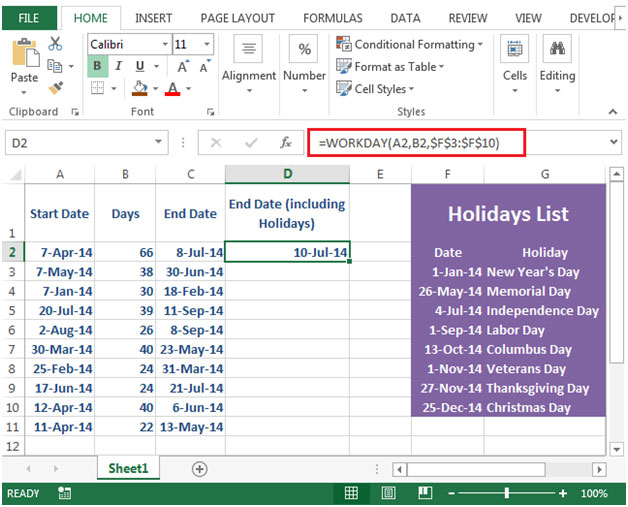• By copying down the formula in below range, we will get the desired output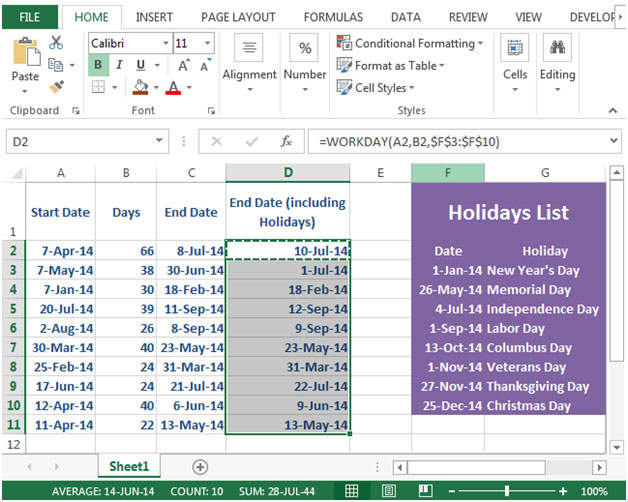You will notice the difference in end date in column C & D. There is a two days gap in output because of the introduction of the third argument (holidays). The holidays that are impacting the calculation are 26-May-14 Memorial Day& 4-Jul-14 Independence Day.

In this way, we can use workday function as a formula of productivity.

1.please help me how to find out end date and time as my working 8 hours i have start date-time 16/4/2020 9:30 then add 16 hour calculate end date and time
16/4/2020 9:30 16 hours end date time ?

•Hi Jitendra,

2.